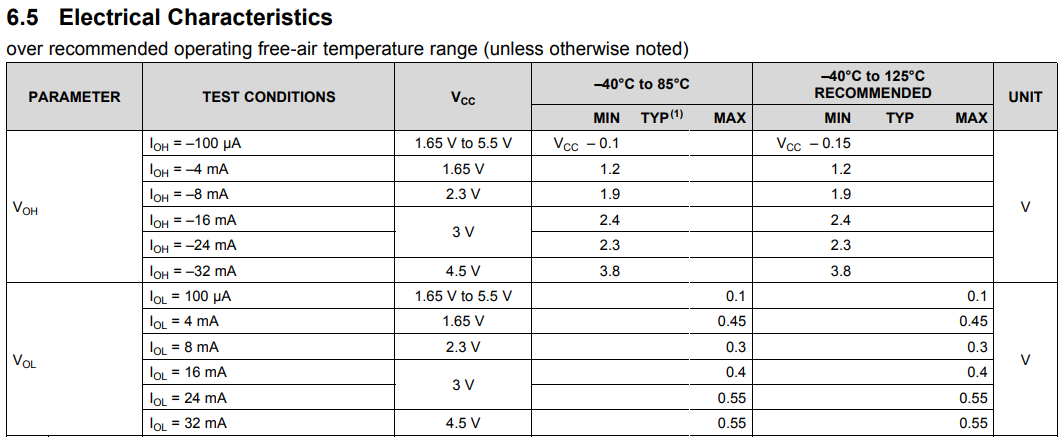If you have a related question, please click the "Ask a related question" button in the top right corner. The newly created question will be automatically linked to this question.

# [FAQ] What is the output transition rate for a logic device?

Other Parts Discussed in Thread: SN74LVC1G08

FAQ: Logic and Voltage Translation > Output Parameters >> Current FAQ

Very few logic devices specify an output transition rate, and those that do only specify it under very specific conditions.

The best method to determine the output transition rate is to measure it using the real device under the same conditions that your system will operate.

The next best method is to use a signal integrity simulator (such as Hyperlynx or ADS) together with an IBIS model and your system's parameters to simulate the output operation. This can give a very accurate representation for the operation of the device under the given system configuration.

The least accurate, but also helpful method is to calculate the value from the given datasheet specifications. This value can easily be calculated if you know the output drive strength of the device (given in the datasheet) and the load capacitance of your system.

Here's an example:

The maximum output low resistance (ROL) can be calculated from the output low voltage (VOL) and the output low current (IOL). Also, the maximum output high resistance (ROH) can be calculated from the output high voltage (VOH) and the output high current (IOH) Here's an example from the SN74LVC1G08 datasheet:Using Ohm's law (V = I*R) and the values for 3V operation (aka 3.3V worst case operation)

ROL = VOL/IOL = 0.55/0.024 = 22.917 Ω

ROH = (VCC-VOH)/IOH = (3-2.3)/.024 = 29.167 Ω

Assuming a load of 50 pF, we can precisely calculate the maximum output transition time using the RC charge/discharge equations: Vc(t) = Vcc*(1-exp(-t/(RC)) , Vc(t) = Vcc*exp(-t/(RC)) -- I'll leave that as an exercise for you.

You can also estimate the transition time as 2*R*C, which is much faster and easier (2 time constants is a charge of ~86.5%, and transition time is measured from 10% to 90% typically):

tLH(max) = 29.167 Ω * 50 pF * 2 = 2.9 ns

tHL(max) = 22.917 Ω * 50 pF * 2 = 2.3 ns

Maximum output transition rate is typically taken as the maximum of these two values, so:

tt = 2.9 ns